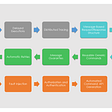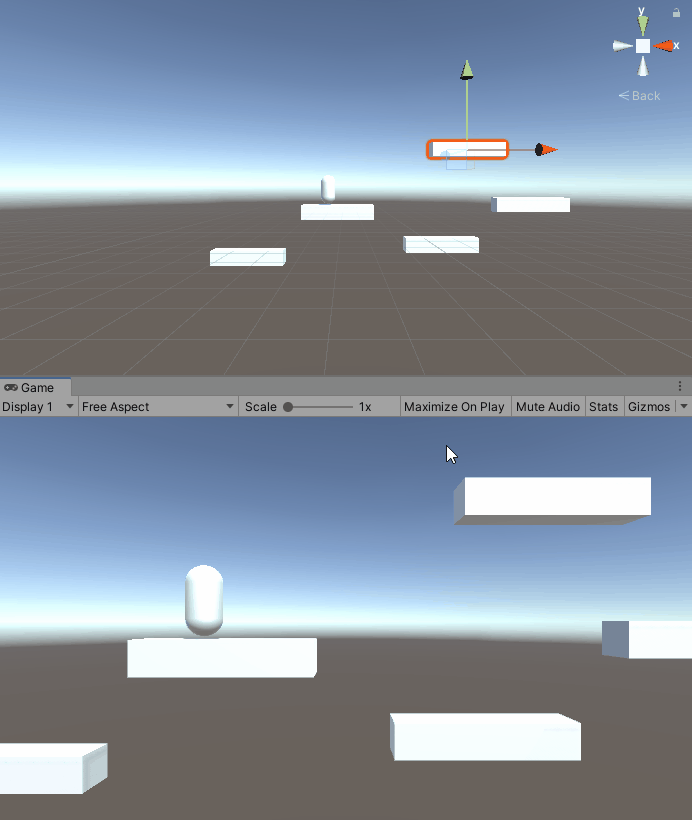# Dynamic Programming On Grids

In this article, we will be solving problems such as finding the least expensive path in a 2d matrix to reach a point, number of ways to reach a position using dynamic programming.

· When a Cost Matrix is given, find the least expensive path in a grid.

· Counting the number of ways to get from one point to another in the provided directions.

Finding Least Expensive Path

Assumption: All costs are positive

Problem Statement: Find a minimum-cost path from cell (0,0) to cell (x,y) given a cost matrix Cost[][], where Cost[i][j] specifies the cost of visiting cell with coordinates (i,j). (All costs are positive integers, we suppose.)

To solve this, it is simple to see that to reach a grid location (i,j), you must have come from one cell above, i.e. (i-1,j), or one cell to your left, i.e. (i,j-1). This indicates that the following recurrence relation will determine the cost of visiting cell (i,j):

Therefore Reccurence Relation :

MinCost(i,j) = min(MinCost(i-1,j),MinCost(i,j-1)) + Cost[i][j]

Now Solving for base Cases :

The difficulty of determining the lowest-cost path is nearly solved now. The basic cases’ values, the topmost row and the leftmost column, are now computed. A cell in the topmost row can only be accessed from the cell to its left. In the case of a zero-based index

`MinCost(0,j) = MinCost(0,j-1) + Cost[j]`

Cost of getting to cell (0,j) = Cost of getting to cell (0,j-1) + Cost of going to cell (0,j) (0,j) Similarly,

`MinCost(i,0) = MinCost(i-1,0) + Cost[i]#include <bits/stdc++.h>using namespace std;#define F(i,a,b) for(int i = (int)(a); i <= (int)(b); i++)#define RF(i,a,b) for(int i = (int)(a); i >= (int)(b); i--)int main(){    int X,Y; //X:number of rows, Y: number of columns    X = Y = 10; //assuming 10X10 matrix    int Cost[X][Y];    F(i,0,X-1)    {        F(j,0,Y-1)        {            //Take input the cost of visiting cell (i,j)            cin>>Cost[i][j];        }    }    int MinCost[X][Y]; //declare the minCost matrix    MinCost = Cost;    // initialize first row of MinCost matrix    F(j,1,Y-1)        MinCost[j] = MinCost[j-1] + Cost[j];    //Initialize first column of MinCost Matrix    F(i,1,X-1)        MinCost[i] = MinCost[i-1] + Cost[i];    //This bottom-up approach ensures that all the sub-problems needed    // have already been calculated.    F(i,1,X-1)    {        F(j,1,Y-1)        {            //Calculate cost of visiting (i,j) using the            //recurrence relation discussed above            MinCost[i][j] = min(MinCost[i-1][j],MinCost[i][j-1]) + Cost[i][j];        }    }    cout<<"Minimum cost from (0,0) to (X,Y) is "<<MinCost[X-1][Y-1];    return 0;}`

Counting the number of ways to get from a starting point to a destination-

Problem: Given a matrix with M rows and N columns, to find the number of ways to reach cell i,j from starting (0,0) given restrictions that one can travel only one cell down or right.

We can relate this problem with the previous one to find the recurrence relation for this problem,

we can find the number of ways to solve this problem for a given (I,j) cell by summing the number of ways of one left and one cell above that is numofways[i-1][j] and numofways[i][j-1].

Therefore Reccurence Relation

numWays(i,j) = numWays(i-1,j) + numWays(i,j-1)

Now, for base case for first row number of ways of reaching a cell is 1, since you can come from one side only and similarly for first column one can come down only in one way.

The answer will be at the last cell of matrix that is numWays[n-1][m-1]

`#include <bits/stdc++.h>using namespace std;#define F(i,a,b) for(int i = (int)(a); i <= (int)(b); i++)#define RF(i,a,b) for(int i = (int)(a); i >= (int)(b); i--)int main(){    int X,Y; //X:number of rows, Y: number of columns    X = Y = 10; //assuming 10X10 matrix    int NumWays[X][Y]; //declare the NumWays matrix    NumWays = 1;    // initialize first row of NumWays matrix    F(j,1,Y-1)        NumWays[j] = 1;    //Initialize first column of NumWays Matrix    F(i,1,X-1)        NumWays[i] = 1;    //This bottom-up approach ensures that all the sub-problems needed    // have already been calculated.    F(i,1,X-1)    {        F(j,1,Y-1)        {            //Calculate number of ways visiting (i,j) using the            //recurrence relation discussed above            NumWays[i][j] = NumWays[i-1][j] + NumWays[i][j-1];        }    }    cout<<"Number of ways from(0,0) to (X,Y) is "<<NumWays[X-1][Y-1];    return 0;}`

The above two were dynamic programming problems since both of these satisfied the following two properties –

Optimal Sub-Structure — Optimal solutions to a problem involves solutions optimal solutions to sub-problem

Overlapping Sub-Problems- Subproblems once computed should be stored in the table for re-use.

Lets talk about on a variation of the first these problems if moving diagonally was allowed then the

Reccurence relation for the above problems would be –

1) MinCost(I,j) = min(MinCost(i-1,j),MinCost[i][j-1],MinCost[i-1][j-1]) + Cost[I,j]

2) numWays(I,j) = numWays(i-1,j) + numWays(i-1,j-1) + numWays(i,j-1)

The intention was to give intuition to problems involving dynamic programming on grids, hope it was helpful !

--

--

--

Love podcasts or audiobooks? Learn on the go with our new app.

## Git Merge vs Rebase## An Opinionated Approach to Developing Event-Driven Microservice Applications with Kafka and…## Install Rancher K3s on Raspberry Pi Cluster## Making Notes in Lark Docs (飞书)## Create shared understanding with ‘What, So What, Now What’## Container Orchestration Platforms## Managing Time with CPU Real-Time Scheduling Algorithm## Inserting values to Binary Search Tree## Understanding the fundamental Linked List Data Structure